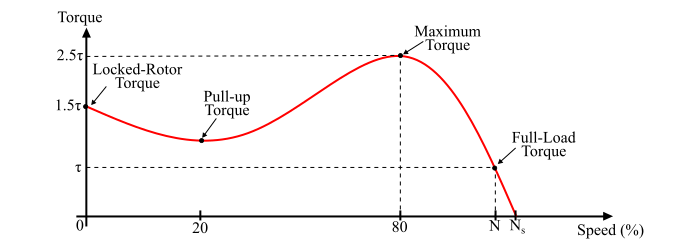# Three-Phase Induction Motor Torque-Speed Characteristics

The torque-speed characteristics of a 3-phase induction motor is defined as the curve plotted between torque developed and rotational speed of the motor. It gives the information about variation in the motor torque with the change in its speed.

As the torque of three-phase induction depends upon its speed but the relationship between them cannot be expressed by a simple equation. Therefore, we use the torque-speed curve to express the relationship between them.## Refer the torque-speed curve, it reveals the following facts −

• If the full-load torque is τ, then the starting torque or locked rotor torque is 1.5 times of τ and the maximum torque (also known as breakdown torque) is 2.5 times of τ.

• The full load speed of the motor is N. If the mechanical load on the shaft is increased, the motor speed will decrease until the electromagnetic torque (or motor torque) is again equal to the load torque. As soon as the two torques are equal, the motor will run at a constant speed but lower than the previous speed. Although, if the torque exceeds the breakdown torque (2.5τ), the will suddenly stop.

• The torque-speed characteristics of a three-phase induction motor is a straight line between the no-load and full-load operating points. The slope of the curve line depends upon the resistance of the rotor circuit i.e. the higher the rotor circuit resistance, the sharper the slope of the curve.

• The small three-phase induction motors (below 10 kW rating) develop their maximum torque at a speed about 80% of synchronous speed whereas large motors (more than 1000 kW rating) develop their maximum torque at a speed about 98% of synchronous speed.